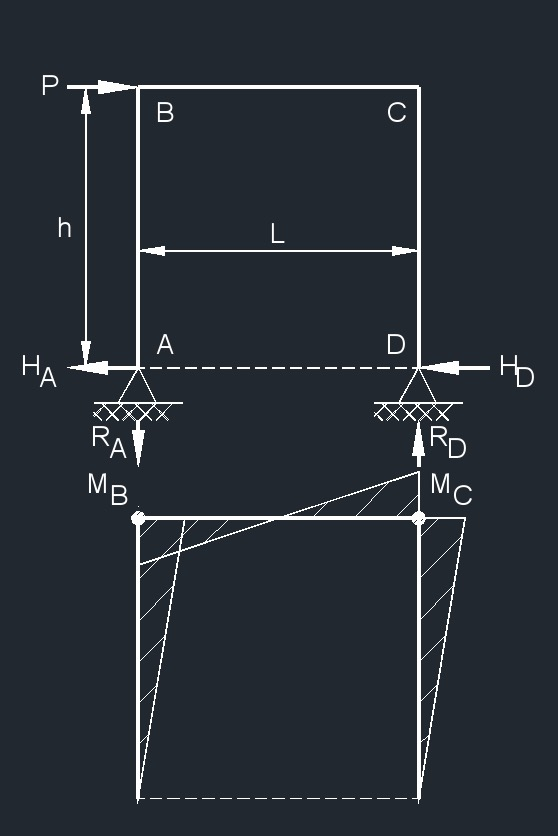# Three Member Frame - Pin/Pin Top Horizontal Point Load

Written by Jerry Ratzlaff on . Posted in Structural

##formulas that use Three Member Frame - Pin/Pin Top Horizontal Point Load

 $$\large{ R_A = R_D = \frac{P\;h}{L} }$$ $$\large{ H_A = H_D = \frac{P}{2} }$$ $$\large{ M_B = M_C = \frac{P\;h}{2} }$$

### Where:

$$\large{ h }$$ = height of frame

$$\large{ H }$$ =  horizontal reaction load at bearing point

$$\large{ M }$$ = maximum bending moment

$$\large{ A, B, C, D }$$ = points of intersection on frame

$$\large{ R }$$ = reaction load at bearing point

$$\large{ L }$$ = span length of the bending member

$$\large{ P }$$ = total concentrated load